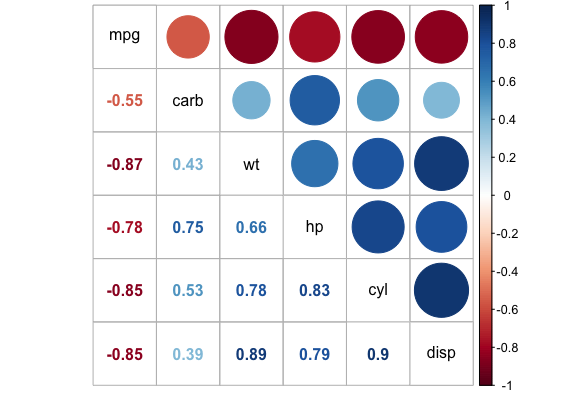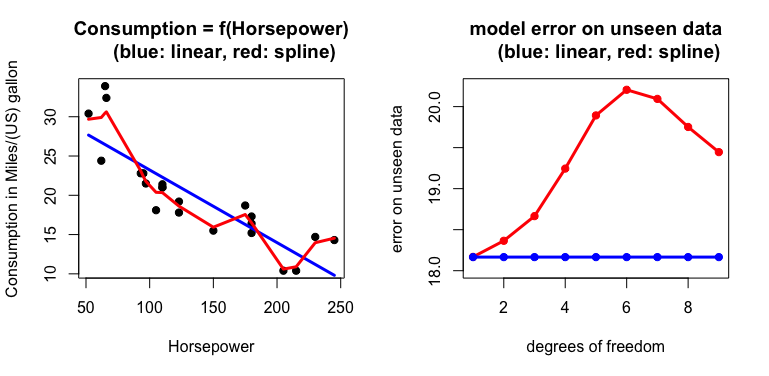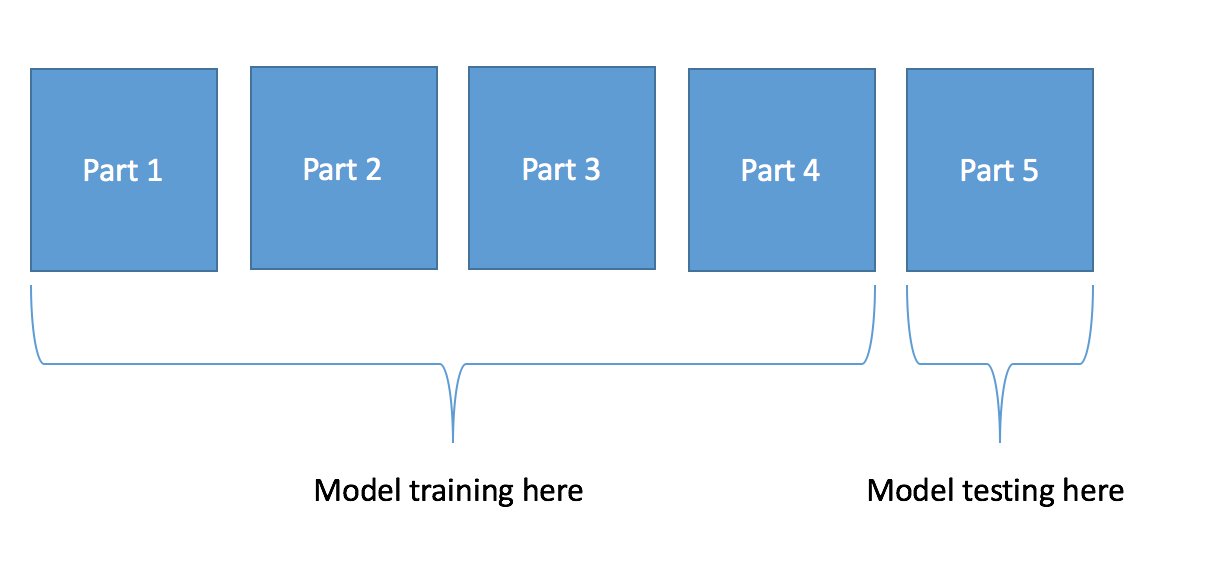Model calibration in the context of this post, is about finding optimal hyperparameters for Statistical/Machine learning (ML) models. Optimal in the sense that they minimize a given criterion such as model’s accuracy on unseen data, model’s precision, Root Mean Squared Error (RMSE), etc. What are ML models’ hyperparameters? Let’s take the example of a linear model:

y = beta_0 + beta_1 x_1 +  beta_2 x_2



Imagine that y is a car’s fuel consumption in Miles/(US) gallon. x_1 is its horsepower, and x_2 its number of cylinders. Knowing the values of x_1 and x_2, we would like to estimate the average value of y for many different cars. beta_1 and beta_2 are unknown model parameters, typically estimated by minimizing the distance between the observed car’s consumption y, and the model beta_0 + beta_1 x_1 + beta_2 x_2. With such a model, we can obtain for example that:

estimated fuel cons. = 0.1 + 0.4 x horsepower +  0.7 x no. of cylinders



Sometimes, when designing our linear model, we will want the unknown coefficients beta_1 and beta_2 to be bounded (beta_1 and beta_2 could otherwise exhibit a high variance). Or, we could want to consider a different polynomial degree d for x_1 or x_2. Whereas beta_1 are beta_2 are model parameters, the polynomial degree d on explanatory variables and the bound s put on parameters beta_1 and beta_2 are model hyperparameters.

Hyperparameters are those parameters that you can tune, in order to increase/decrease the model’s performance. d is a degree of freedom. It controls model’s flexibility. The higher d, the more flexible our model - meaning than it could almost fit “anything”. s is a regularization parameter that stabilizes model estimates. Increasing d might lead to overfitting, and a lower d, to underfitting. Overfitting or underfitting are about: too much flexibility or not enough. We’ll use the mtcars dataset to illustrate these concepts. This dataset is available from R console, as:

data(mtcars)


According to its description, mtcars is extracted from 1974 Motor Trend US magazine, and comprises fuel consumption (in Miles/(US) gallon) and 10 aspects of automobile design and performance for 32 automobiles (1973–74 models). We’ll use 5 explanatory variables among 10 here.

mpg:	Miles/(US) gallon # this is y
cyl:	Number of cylinders # this is x_1
disp:	Displacement (cu.in.) # this is x_2
hp:     Gross horsepower # this is x_3
wt:     Weight (1000 lbs) # this is x_4
carb:	Number of carburetors # this is x_5


Below, are the correlations between the target variable (to be explained), mpg, and explanatory variables x_1, …, x_5. We use R package corrplot to plot these correlations.All the explanatory variables are negatively correlated to the fuel consumption (in Miles/(US) gallon). A marginal increase in any of them leads, on average, to a decrease in fuel consumption. Now, in order to illustrate the concepts of overfitting and underfitting, we fit a linear model and a smoothing spline to mpg (consumption) and hp (horsepower).On the left: model fitting on 23 cars, for a linear model and a spline. The linear model fits all the points parsimoniously, but the spline tries to memorize the patterns. On the right: errors obtained by each model on the 9 remaining cars, as a function of the spline’s degrees of freedom. That’s overfitting, illustrated. In other situations, a linear model can also fit (very) poorly, because it’s not flexible enough.

So, how do we find a good compromise between overfitting or underfitting? One way to achieve it is to use a hold-out sample, as we did on the previous example - with 23 cars out of 32 in the training procedure, and 9 for testing. Another way is to use cross-validation. The idea of cross-validation is to divide the whole dataset into k parts (usually called folds); each part being successively included into a training set or a testing set.On this graph, we have k=5. crossval is a - work in progress - R package, for doing just that. WHY did I implement it? Because R models are contributed by many different people. So, you’re not using a unified interface when training them. For example, in order to obtain predictions for 2 different models, you can have 2 different specifications of function predict:

predict(fitting_obj_model1, newx)


or

predict(fitting_obj_model2, newdata)


fitting_obj_model* are the trained models 1 and 2. newx and newdata are the unseen data on which we would like to test the trained model. The position of arguments in function calls do also matter a lot. Idea: use a common cross-validation interface for many different models. Hence, crossval. There is still room for improvement. If you find cases that are not covered by crossval, you can contribute them here. Currently, the package can be installed from Github as (in R console):

library(devtools)
devtools::install_github("thierrymoudiki/crossval")


Here is an example of use of crossval applied to glmnet (with my old school R syntax yeah, I like it!):

require(glmnet)
require(Matrix)

data("mtcars")
df <- mtcars[, c(1, 2, 3, 4, 6, 11)]
summary(df)

# create response and explanatory variables
X <- as.matrix(df[, -1])
y <- df\$mpg

# grid of model hyperparameters
tuning_grid <- expand.grid(alpha = c(0, 0.5, 1),
lambda = c(0.01, 0.1, 1))
n_params <- nrow(tuning_grid)

# list of cross-validation results
# - 5-fold cross-validation (k)
# - repeated 3 times (repeats)
# - cross-validation on 80% of the data (p)
# - validation on the remaining 20%
cv_results <- lapply(1:n_params,
function(i)
crossval::crossval(
x = X,
y = y,
k = 5,
repeats = 3,
p = 0.8,
fit_func = glmnet::glmnet,
predict_func = predict.glmnet,
packages = c("glmnet", "Matrix"),
fit_params = list(alpha = tuning_grid[i, "alpha"],
lambda = tuning_grid[i, "lambda"])
))
names(cv_results) <- paste0("params_set", 1:n_params)
print(cv_results)



Many other examples of use of the package can be found in the README. Also, R packages like caret or mlr do similar things, but with a different philosophy. You may want to try them out too.

Note: I am currently looking for a gig. You can hire me on Malt or send me an email: thierry dot moudiki at pm dot me. I can do descriptive statistics, data preparation, feature engineering, model calibration, training and validation, and model outputs’ interpretation. I am fluent in Python, R, SQL, Microsoft Excel, Visual Basic (among others) and French. My résumé? Here!